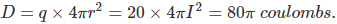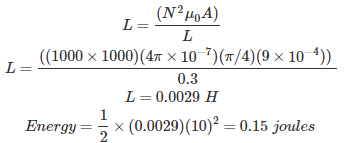# MCQs on Electromagnetic Fields

##### Page 3 of 20. Go to page 1 2 3 4 5 6 7 8 9 10 11 12 13 14 15 16 17 18 19 20
01․ The drawback of Daltons atomic structure is that, it says
the atoms can neither be created nor be destroyed.
unique nature of atom.
most of the volume of an atom is empty space.
none of these.

The Dalton’s atomic theory deals with chemical reactions. During chemical reactions, an atom can neither be created nor be destroyed. But, in radio active reaction, new atoms can be created by dividing other atoms. Thus, this is the very big drawback of this method.

02․ According to Coulomb’s law, the force of attraction or repulsion, between to electrical charges is
directly proportional to the square of the distance between them.
inversely proportional to the square of the distance between them.
inversely proportional to the distance between them.
directly proportional to the distance between them.

Coulomb’s law states that the force of attraction or repulsion, F between two charges q1 and q2 coulombs, connected at two different points in a medium, is directly proportional to the product of their magnitude and inversely proportional to the square of distance r between them.

03․ Ohm’s law in point from field theory can be expressed as
J = σE.
V = IR.
J = E / σ.
R = ρ × ( l / A).

In linear medium, the conduction current density is proportional to the applied electric field: J = σE = E / ρ [ A / m2] where, σρ = 1 / r [ S / m ( Siemens / meter ) ] is the conductivity and ρr [ Ohm - meters] is the resistivity of the material. This equation is clearly related to the Ohm’s law of circuit theory; in field theory, it is called the point form of Ohm’s law.

04․ A metal surface with 1 meter radius and surface charge density of 20 coulombs / m2 is enclosed in a 10 m side cube. The total outward electric displacement normal to the surface of the cube is
40π coulombs.
80π coulombs.
10π coulombs.
none of these.05․ Inside a hollow conducting sphere
electric field is zero.
electric field is non zero constant.
electric field changes with magnitude of the charge.
electric field changes with distance from the center.

According to the Gauss Law, for any concentric sphere, inside conducting sphere, the total charge enclosed is zero hence electric field inside is zero.

06․ A long wire composed of a smooth round conductor runs above and parallel to the ground (assumed to be a large conducting plane). A high voltage exists between the conductor and the ground. The maximum electric stress occurs at
lower surface of the conductor.
upper surface of the conductor.
the ground surface.
midway between the conductor and ground.

The maximum electric stress occurs at lower surface of the conductor because of maximum field intensity.

07․ The energy stored in the magnetic field in a solenoid 30 cm long and 3 cm diameter wound with 1000 turns of wire carrying a current at 10 amp, is
0.015 joule.
0.15 joule.
0.015 joule.
1.15 joule.08․ Midway between two equal and similar charges, a third equal and similar charge is placed, then this third charge will
remain in stable equilibrium.
be in unstable equilibrium.
not be equilibrium.
will move out of the field of influence of two charges.

Force on the first two charges is cancelled out due to the presence of third charge. Hence, the system remains in stable equilibrium.

09․ It was suggested by _____________ that the electric field should be imagined to be divided into tubes of force containing a fixed number of line of force
Kelvin.
Newton.
all of above.

Faraday is the only person who did this work among all the above three persons. He assumed these tubes to the elastic and having the property of contracting longitudinally that repels laterally. With the help of this property, he has given the above statement.

10․ Electric flux in coulombs emanating from any surface of a cube containing C coulombs of charge at its centre is
Q / 4π.
4πQ / 6ε.
Q / 6.
Q / 6ε.

Gauss’s Law says that the total flux through a closed surface is equal to the enclosed charge q over ε0. The particle is located at the center, so because of symmetry, the electric field and thus the flux is the same on any face. So, the flux through a single face as well as center path is Q / 6ε.

<<<12345>>>